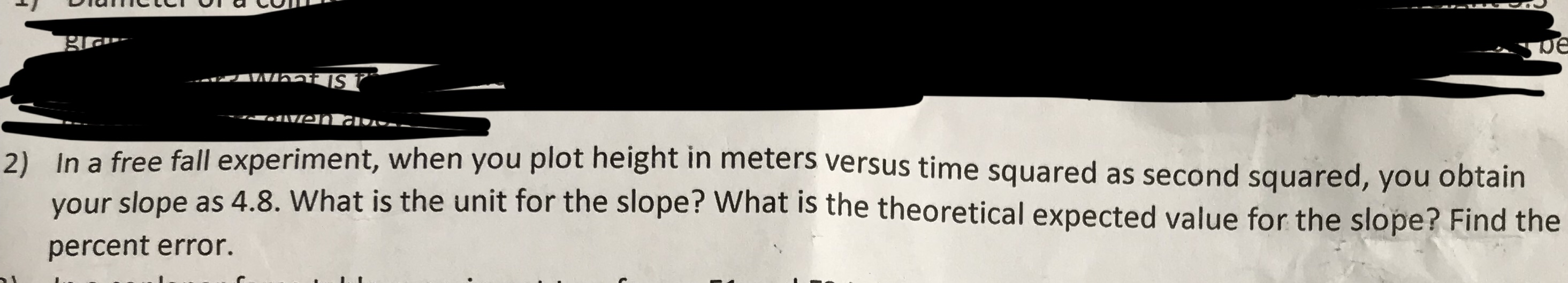# In a free fall experiment, when you plot height in meters versus time squared as second s your slope as 4.8. Wh percent error. 2) quared, you obtain at is the unit for the slope? What is the theoretical expected value for the slope? Find the

Questionhelp_outlineImage TranscriptioncloseIn a free fall experiment, when you plot height in meters versus time squared as second s your slope as 4.8. Wh percent error. 2) quared, you obtain at is the unit for the slope? What is the theoretical expected value for the slope? Find the fullscreen

### Want to see the step-by-step answer?